# Mackey topology

(diff) ← Older revision | Latest revision (diff) | Newer revision → (diff)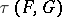on a space, being in duality with a space(over the same field)
The topology of uniform convergence on the convex balanced subsets ofthat are compact in the weak topology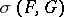(defined by the duality betweenand). It was introduced by G.W. Mackey . The Mackey topology is the strongest of the separated locally convex topologies (cf. Locally convex topology) which are compatible with the duality betweenand(that is, separated locally convex topologies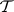onsuch that the set of all continuous linear functionals onendowed with the topologycoincides with). The families of sets inwhich are bounded relative to the Mackey topology and bounded relative to the weak topology coincide. A convex subsets ofis equicontinuous whenis endowed with the Mackey topology if and only if it is relatively compact in the weak topology. If a separated locally convex spaceis barrelled or bornological (in particular, metrizable) and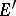is its dual, then the Mackey topology on(being dual with) coincides with the initial topology on. For pairs of spaces () in duality the Mackey topologyis not necessarily barrelled or metrizable. A weakly-continuous linear mapping of a separated locally convex spaceinto a separated locally convex spaceis continuous relative to the Mackey topologies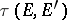and. A locally convex spaceis called a Mackey space if the topology onis. Completions, quotient spaces and metrizable subspaces, products, locally convex direct sums, and inductive limits of families of Mackey spaces are Mackey spaces. Ifis a Mackey space andis a weakly-continuous mapping ofinto a locally convex space, thenis a continuous linear mapping ofinto. Ifis a quasi-complete Mackey space and the space dual toequipped with the strong-topology is semi-reflexive, thenis reflexive.